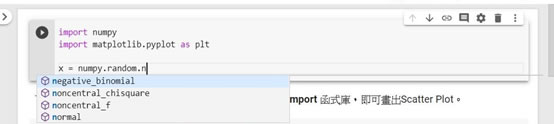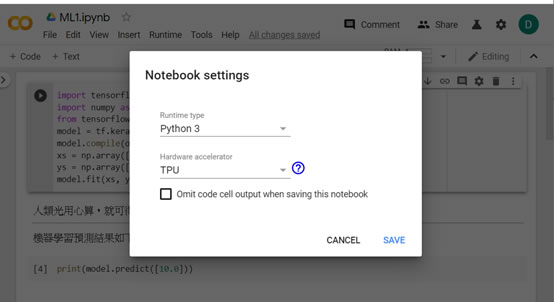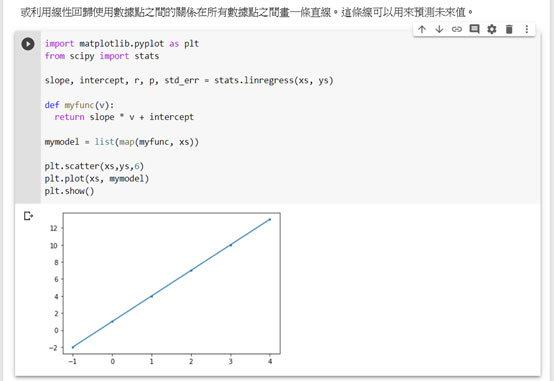ISSN 2077-8813

## 首頁 >技術論壇

Colab目的在提供Machine Learning機器學習教育訓練及研究用，不須下載、不須安裝就可直接應用Python 2.7 與 Python 3.6資源庫，對初學者來說可以快速入門，不需耗時間在環境設定上。程式碼預設會直接儲存在開發者的Google Drive雲端硬碟中，執行時由虛擬機提供強大的運算能力，不會用到本機的資源。但要注意在閒置一段時間後，虛擬機會被停止並回收運算資源，此時只需再重新連接即可。接下來我們就來看操作環境：

Colab Notebook環境介紹import numpy
import matplotlib.pyplot as plt

x = numpy.random.normal(5.0, 1.0, 100000)

plt.hist(x, 100)
plt.show()y = numpy.random.normal(10.0, 2.0, 1000)

plt.scatter(x, y)
plt.show()，方便我們查詢網站stack overflow的相關解答，非常實用。修改後就可畫出正確分布圖形了：drive.mount('/gdrive')with open('/gdrive/My Drive/foo.txt', 'w') as f:
!cat '/gdrive/My Drive/foo.txt'# Import PyDrive and associated libraries.
# This only needs to be done once in a notebook.

# Authenticate and create the PyDrive client.
# This only needs to be done once in a notebook.
auth.authenticate_user()

# Create & upload a text file.

# List .txt files in the root.
# Search query reference:
listed = drive.ListFile({'q': "title contains '.txt' and 'root' in parents"}).GetList()
for file in listed:
print('title {}, date {}, id {}'.format(file['title'], file['createdDate'], file['id']))# A file ID looks like: laggVyWshwcyP6kEI-y_W3P8D26sz
file_id = '填入你自己的file ID'import tensorflow as tf
import numpy as np
from tensorflow import keras

model = tf.keras.Sequential([keras.layers.Dense(units=1, input_shape=)])
model.compile(optimizer='sgd', loss='mean_squared_error')

xs = np.array([-1.0, 0.0, 1.0, 2.0, 3.0, 4.0], dtype=float)
ys = np.array([-2.0, 1.0, 4.0, 7.0, 10.0, 13.0], dtype=float)
# 以上code定義此neural network

model.fit(xs, ys, epochs=100)

import matplotlib.pyplot as plt
from scipy import stats
slope, intercept, r, p, std_err = stats.linregress(xs, ys)

def myfunc(v):
return slope * v + intercept

mymodel = list(map(myfunc, xs))
plt.scatter(xs,ys,6)
plt.plot(xs, mymodel)
plt.show()print(model.predict([10.0]))• 芬蘭在2018年推出免費的AI線上課程，目的是教育公民有關新技術的知識。本課程涵蓋AI哲學含意到貝氏概率（Bayesian probability）技術在內，完全沒有程式碼，只需統計學知識。 https://www.elementsofai.com/
• https://jupyter.org/ Jupyter Notebook
• Learn TensorFlow 1: The "Hello World" of machine learning
• 機器學習